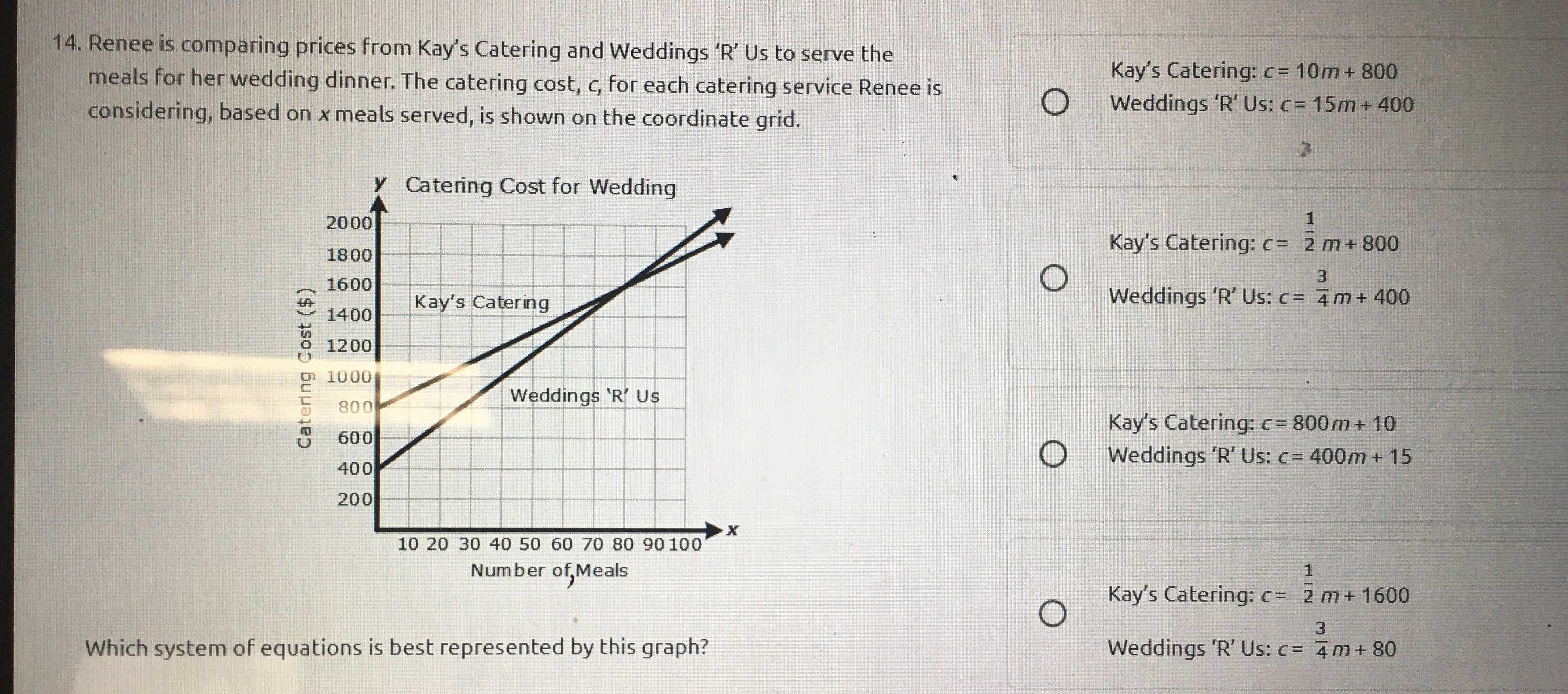### ¿Todavía tienes preguntas de matemáticas?

Pregunte a nuestros tutores expertos
Algebra
Pregunta14. Renee is comparing prices from Kay's Catering and Weddings ' $$R$$ ' Us to serve the meals for her wedding dinner. The catering cost, $$c$$ , for each catering service Renee is considering, based on $$x$$ meals served, is shown on the coordinate grid.

A. Kay's Catering: $$c = 10 m + 800$$

Weddings 'R' Us: $$c = 15 m + 400$$

B. Kay's Catering: $$c = \frac { 1 } { 2 } m + 800$$

Weddings 'R' Us: $$c = \frac { 3 } { 4 } m + 400$$

C. Kay's Catering: $$c = 800 m + 10$$

Weddings 'R' Us: $$c = 400 m + 15$$

D. Kay's Catering: $$c = \frac { 1 } { 2 } m + 1600$$

Weddings 'R' Us: $$c = \frac { 3 } { 4 } m + 80$$ A.